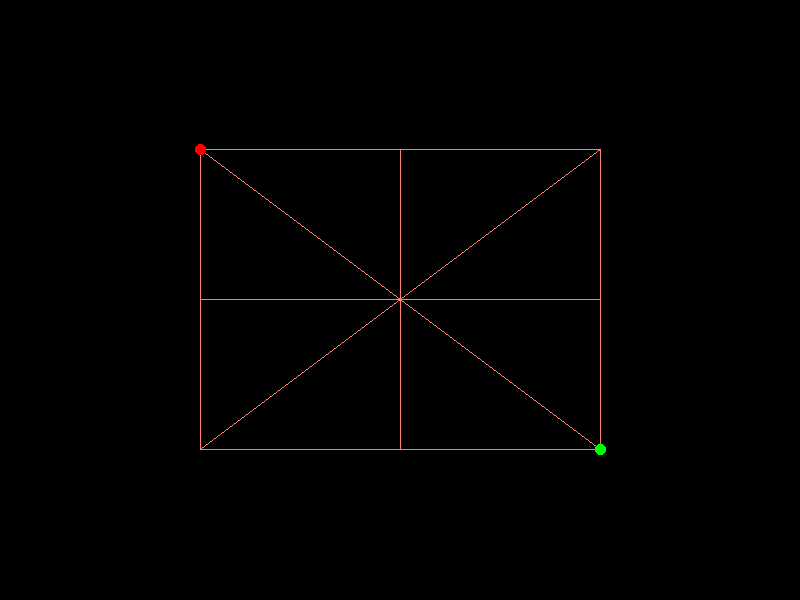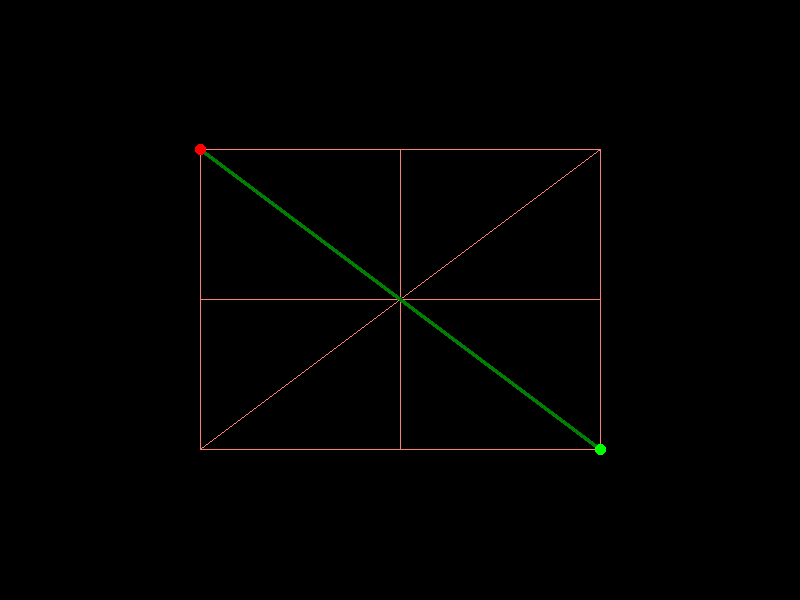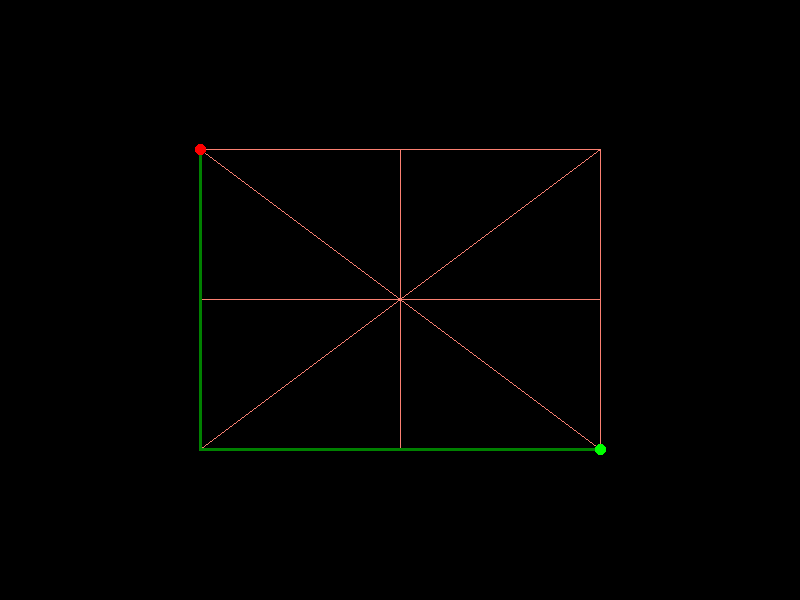# Pathfinding#

Xarray-spatial’s Pathfinding provides a comprehensive tool for finding the shortest path from one point to another in a raster that can contain any level of complex boundaries or obstacles amidst an interconnected set of traversable path segments.

## Importing Packages#

:

import numpy as np
import pandas as pd

import xrspatial


To download the examples data, run the command xrspatial examples in your terminal. All the data will be stored in your current directory inside a folder named xrspatial-examples.

## A*#

A* is an informed search algorithm, or a best-first search, meaning that it is formulated in terms of weighted graphs: starting from a specific starting node of a graph, it aims to find a path to the given goal node having the smallest cost (min distance travelled, shortest time, …).

The xrspatial.a_star_search function calculates the shortest path in pixel space from a start location to a goal location through a given aggregate surface graph. The graph should be a line raster which contains crossable and non-crossable (a.k.a walls or barrieres) values. Note that both start and goal are in (lon, lat), or (x, y) coordinate space and must be within the graph. xrspatial.a_star_search provides 2 separate options, snap_start and snap_goal, which can be set to true to snap locations to the nearest valid value before beginning pathfinding. It also provides a connectivity option to indicate neighborhood structure. This value can be set to 4 or 8 for 4-connectivity or 8-connectivity.

Let’s generate a fake line raster and find the shortest path with A*.

• First, we’ll generate a line raster by setting up a pandas DataFrame specifying the line coordinates.

• Then, we’ll aggregate that into a lines raster with Canvas.line

• Once we have that, we’ll choose a start and goal point to put into the a* pathfinding function.

• For visualization, we’ll also aggregate those points and render them in an image together with the lines.

:

from xrspatial import a_star_search

# define range of x and y
xrange = (0, 4)
yrange = (0, 4)

# create line raster
ys = [1, 1, 3, 3, 1, 1, np.nan, 1, 3, np.nan, 1, 3, np.nan, 1, 3, np.nan, 2, 2]
xs = [1, 3, 3, 1, 1, 3, np.nan, 1, 3, np.nan, 3, 1, np.nan, 2, 2, np.nan, 1, 3]
line_df = pd.DataFrame(dict(x=xs, y=ys))

W = 800
H = 600
cvs = ds.Canvas(plot_width=W, plot_height=H, x_range=xrange, y_range=yrange)
line_agg = cvs.line(line_df, x="x", y="y").astype(int)

# pick up 2 random locations
start = (3, 1)
goal = (1, 3)

start_df = pd.DataFrame({"x": [start], "y": [start]})
start_agg = cvs.points(start_df, "x", "y")

goal_df = pd.DataFrame({"x": [goal], "y": [goal]})
goal_agg = cvs.points(goal_df, "x", "y")


:We’re now ready to apply a_star_search.

### Calculating the 8-connectivity shortest path#

• To calculate the path, we input the line raster and the start and goal point coordinates.

• We also set the barriers; i.e. cells that are not crossable. In our case, any cell with a value of 0 (all the black non-line cells).

• Finally, we’ll also set snap_start and snap_goal to True.

• Note: since a_star_search uses 8-connectivity by default, we don’t need to pass that in.

The shortest path is highlighted in the rendering below.

:

# find the path from start to goal,
# barriers are uncrossable cells. In this case, they are cells with a value of 0

path_agg_8_connectivity = a_star_search(
line_agg, start, goal, barriers=, snap_start=True, snap_goal=True
)


:### 4-connectivity#

For 4-connectivity distance, we use the same arguments as above, but set the connectivity to 4.

:

# find the path from start to goal,
# barriers are uncrossable cells. In this case, they are cells with a value of 0

path_agg_4_connectivity = a_star_search(
line_agg, start, goal, barriers=, snap_start=True, snap_goal=True, connectivity=4
)


: